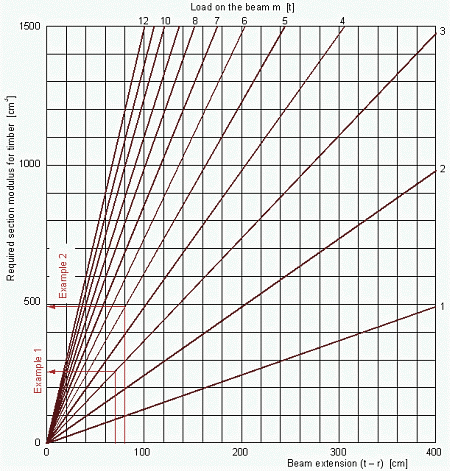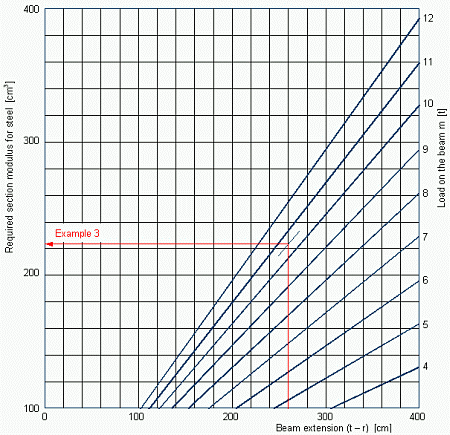4.3   Beam-diagramsThe solutions of equation (11) for timber beams are presented graphically in Figure 16. The load m varies between 1 and 12 t, and the extension of the beam (t - r) varies between 0 and 400 cm. With these entries all relevant cases may be covered. It becomes clear however, that for coils of the weight class XH, where the beam length t must be greater than 2.7 m according to Figure 13 and hence the expected beam extension (t - r) greater than 1.5 m, a proper load distribution with timber beams cannot be obtained with reasonable expense. Therefore, a similar diagram in Figure 17 shows the solutions of equation (12) for steel beams.Figure 16: Required section modulus for timber beam bedding Example 1: A coil of 6 t mass on skids of 0.9 m length of timbers is placed on two longitudinal beams of 1.6 m length. The beam extension (t - r) is 70 cm. Each beam is loaded with 3 t. The diagram in Figure 16 shows a required section modulus of about 260 cm³. Hence, beams of 12 x 12 cm are necessary (see Table 1). Example 2: A coil of 10 t mass on skids of 1.0 m length of timbers is placed on two longitudinal beams of 1.8 m length. The beam extension (t - r) is 80 cm. Each beam is loaded with 5 t. The diagram in Figure 16 shows a required section modulus of about 490 cm³. Hence, beams of 15 x 15 cm are just sufficient (see Table 1). Example 3: A coil of 21 t mass and mC/P = 0.75 requires for safeguarding the longitudinal strength of the container two beams of 3.8 m length according to Figure 13. The load of 10.5 t is transferred to each of the beams over a length of about 1.3 m. So the beam extension (t - r) is about 260 cm. With this entry, the diagram in Figure 17 shows a required section modulus of about 225 cm³. With this, steel beams of 14 x 14 cm are just not sufficient (see Table 2). Stronger ones should be used.Figure 17: Required section modulus for steel beam bedding© GDV   |  Container Handbook  |  German versionContact  |  Site Map  |  Glossary  |  Legal Notice